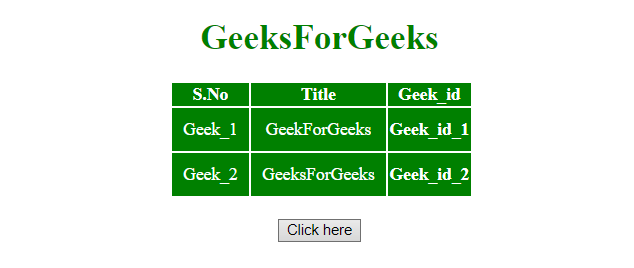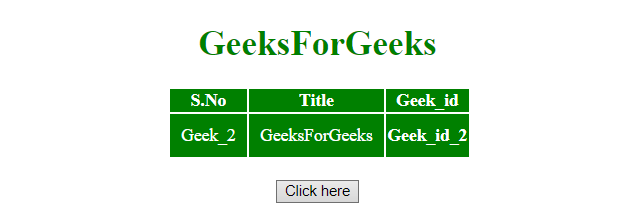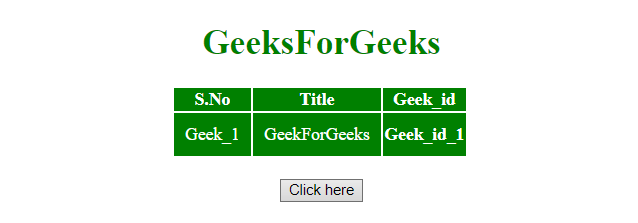Open in App
Not now

# How to remove table row from table using jQuery ?

• Last Updated : 03 Aug, 2021

The jQuery remove() method is used to remove a row from HTML table.
jQuery remove() Method: This method removes the selected elements alongwith text and child nodes. This method also removes data and events of the selected elements.

Syntax:

`\$(selector).remove(selector)`

Parameters: It accepts single parameter selector which is optional. It specifies one or more elements to be removed. If multiple elements are to be removed, separate them with a comma (,) operator.

Example 1: This example first selects the row by id value and then removes it by using remove() method.

 ` ``<``html``> ``    ``<``head``> ``     ` `        ``<``title``> ``            ``How to remove a table row``            ``from table``        `` ``         ` `        ``<``script` `src` `= ``"https://ajax.googleapis.com/ajax/libs/jquery/3.4.0/jquery.min.js"``>``        ```` ` `        ``<``style``>``            ``#myCol {``                ``background:green;``            ``}``            ``table {``                ``color:white;``            ``}``            ``#Geek_p {``                ``color:green;``                ``font-size:30px;``            ``}``            ``td {``                ``padding:10px;``            ``}``        ````    `` ``     ` `    ``<``body``>``        ``<``center``> ``            ``<``h1` `style` `= ``"color:green;"` `> ``                ``GeeksForGeeks ``            `` ``                 ` `            ``<``table``>``                ``<``colgroup``>``                    ``<``col` `id``=``"myCol"``                        ``span``=``"2"``>``                    ``<``col` `style``=``"background-color:green"``>``                ````                 ` `                ``<``tr``>``                    ``<``th``>S.No``                    ``<``th``>Title``                    ``<``th``>Geek_id``                ````                ``<``tr` `id` `= ``"row1"``>``                    ``<``td``>Geek_1``                    ``<``td``>GeekForGeeks``                    ``<``th``>Geek_id_1``                ````                ``<``tr``>``                    ``<``td``>Geek_2``                    ``<``td``>GeeksForGeeks``                    ``<``th``>Geek_id_2``                ````            ````            ``<``br``>``             ` `            ``<``button` `onclick` `= ``"Geeks()"``> ``                ``Click here``            `` ``             ` `            ````            ``<``script``> ``                ``function Geeks() {``                        ``\$("#row1").remove();``                ``}``            ````        ````    `` ``                    `

Output:

• Before clicking on the button:• After clicking on the button:Example 2: This example first select the last <tr> element of its parent and removes it by using remove() method.

 ` ``<``html``> ``    ``<``head``> ``        ``<``title``> ``            ``How to remove table row``            ``from table``        `` ``         ` `        ``<``script` `src` `= ``"https://ajax.googleapis.com/ajax/libs/jquery/3.4.0/jquery.min.js"``>``        ````     ` `        ``<``style``>``            ``#myCol {``                ``background:green;``            ``}``            ``table {``                ``color:white;``            ``}``            ``#Geek_p {``                ``color:green;``                ``font-size:30px;``            ``}``            ``td {``                ``padding:10px;``            ``}``        ````    `` ``     ` `    ``<``body``>``        ``<``center``> ``            ``<``h1` `style` `= ``"color:green;"` `> ``                ``GeeksForGeeks ``            `` ``                 ` `            ``<``table``>``                ``<``colgroup``>``                    ``<``col` `id``=``"myCol"``                        ``span``=``"2"``>``                    ``<``col` `style``=``"background-color:green"``>``                ````                 ` `                ``<``tr``>``                    ``<``th``>S.No``                    ``<``th``>Title``                    ``<``th``>Geek_id``                ````                ``<``tr` `id` `= ``"row1"``>``                    ``<``td``>Geek_1``                    ``<``td``>GeekForGeeks``                    ``<``th``>Geek_id_1``                ````                ``<``tr``>``                    ``<``td``>Geek_2``                    ``<``td``>GeeksForGeeks``                    ``<``th``>Geek_id_2``                ````            ````            ``<``br``>``             ` `            ``<``button` `onclick` `= ``"Geeks()"``> ``                ``Click here``            `` ``             ` `            ``<``script``> ``                ``function Geeks() {``                    ``\$('tr:last-child').remove();``                ``}``            `` ``        ````    `` ``                    `

Output:

• Before clicking on the button:• After clicking on the button:jQuery is an open source JavaScript library that simplifies the interactions between an HTML/CSS document, It is widely famous with it’s philosophy of “Write less, do more”.
You can learn jQuery from the ground up by following this jQuery Tutorial and jQuery Examples.

My Personal Notes arrow_drop_up